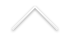The area of mathematical physics has many different branches. In particular, our group's research lies in the intersection between differential geometry and theoretical physics. The scope of the research varies from applications of differential geometric techniques to the study of classical and quantum mechanical systems to applications of physical concepts developed in Quantum Field Theory to solve problems related to differential geometry and topology. Some key words are: Symplectic and Poisson geometry, geometric mechanics, Lie algebroids and groupoids, super-geometry, Topological Field Theories.

Researchers:

• Rolci Cipolatti
• Alejandro Cabrera
• I-Shih Liu
• Anatoli Leontiev